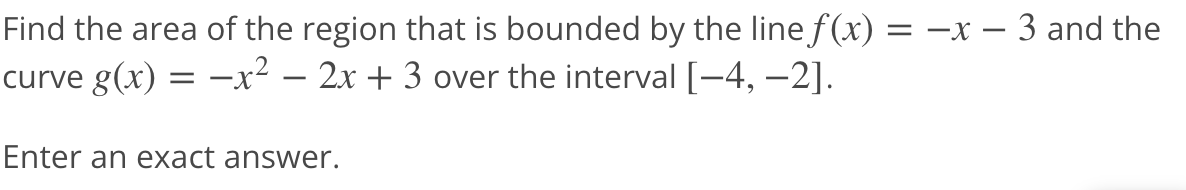# Question Solved1 Answer= = Find the area of the region that is bounded by the line f(x) = -x – 3 and the curve g(x) = -x2 – 2x + 3 over the interval [-4, -2]. = Enter an exact answer.Transcribed Image Text: = = Find the area of the region that is bounded by the line f(x) = -x – 3 and the curve g(x) = -x2 – 2x + 3 over the interval [-4, -2]. = Enter an exact answer.
More
Transcribed Image Text: = = Find the area of the region that is bounded by the line f(x) = -x – 3 and the curve g(x) = -x2 – 2x + 3 over the interval [-4, -2]. = Enter an exact answer.